# Indefinite limits and expressions, evaluations of

(diff) ← Older revision | Latest revision (diff) | Newer revision → (diff)

Methods for computing limits of functions given by formulas that cease to have a meaning when the limiting values of the argument are formally substituted in them, that is, go over into expressions like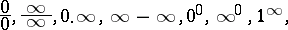for which one cannot judge whether the required limits exist or not without saying anything about finding their values if they exist. The basic instrument of evaluating indeterminacies is Taylor's formula (cf. Taylor formula), by means of which one singles out the principal part of a function. Thus, in the case of an indeterminacy of the type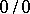, for which in order to find the limit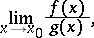whereone represents the functionsandby Taylor's formulas in a neighbourhood of(if this is possible) up to the first non-zero term: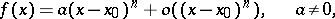as a result one finds that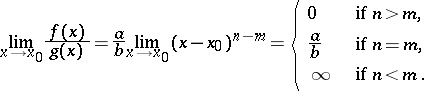In the case of an indeterminacy of the type, in order to find the limitwhere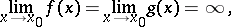one applies the transformationwhich reduces the problem to the evaluation of an indeterminacy of type.

Indeterminacies of the types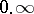orare also conveniently reduced to typeby the following transformations: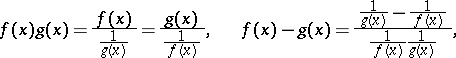respectively.

For evaluating indeterminacies of the types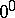,orit is appropriate first to take the logarithm of the expressions whose limits are to be found.

Another general method for evaluating indeterminacies of the typesorand those reducible to them is the l'Hospital rule.